# The function f ( x ) = | x 2 − 4 | x | + 3 | .### Single Variable Calculus: Concepts...

4th Edition
James Stewart
Publisher: Cengage Learning
ISBN: 9781337687805### Single Variable Calculus: Concepts...

4th Edition
James Stewart
Publisher: Cengage Learning
ISBN: 9781337687805

#### Solutions

Chapter 1, Problem 5P
To determine

## To sketch: The function f(x)=|x2−4|x|+3|.

Expert Solution

### Explanation of Solution

Given:

The function is f(x)=|x24|x|+3|.

Result used:

Even function:

A function is even if and only if f(x)=f(x) for all x in the domain of f.

Calculation:

Consider the function f(x)=|x24|x|+3|

Observe that x2=(x)2and |x|=|x|

Therefore, f(x)=f(x) and thus the given function is an even function.

Thus, for all x0.

f(x)=|x24x+3|=(x1)(x3)

The function can be split into four different cases as follows:

Case (i):

For 0x<1,and x3

f1(x)=x24x+3

To sketch: Use the online graphing calculator and draw the graph of the function: f1(x)=x24x+3 as shown below in Figure (1)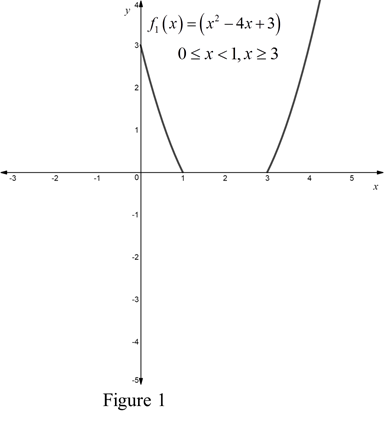Case (ii):

For 1x<3

f1(x)=(x24x+3)

To sketch: Use the online graphing calculator and draw the graph of the function: f1(x)=(x24x+3) as shown below in Figure (2)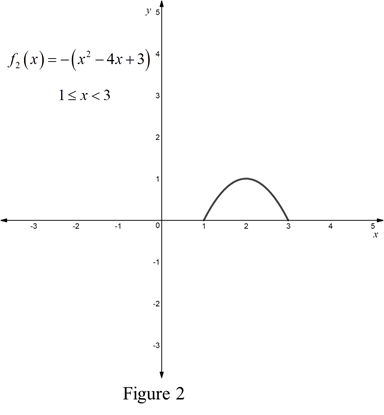Case (iii):

For 1<x0,x3

f1(x)=x2+4x+3

To sketch: Use the online graphing calculator and draw the graph of the function: f1(x)=x2+4x+3 as shown below in Figure (3)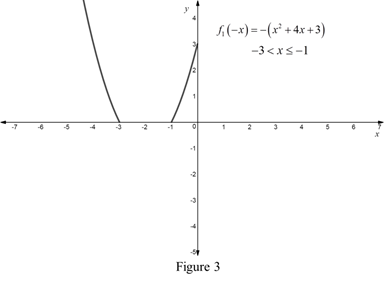Case (iv):

For 3<x1

f2(x)=(x2+4x+3)

To sketch: Use the online graphing calculator and draw the graph of the function: f2(x)=(x2+4x+3) as shown below in Figure (4)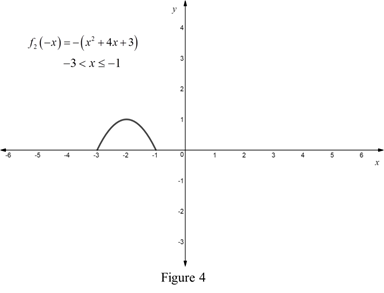Combine all the graphs in figure 1, 2, 3 and 4 as shown below in Figure 5

To sketch: Use the online graphing calculator and draw the graph of the function: f(x)=|x24|x|+3| as shown below in Figure (5)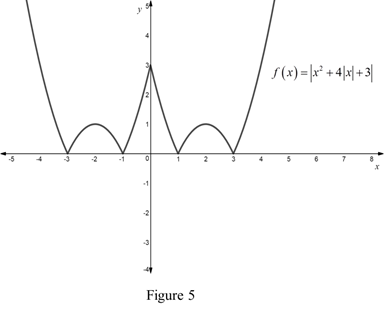### Have a homework question?

Subscribe to bartleby learn! Ask subject matter experts 30 homework questions each month. Plus, you’ll have access to millions of step-by-step textbook answers!